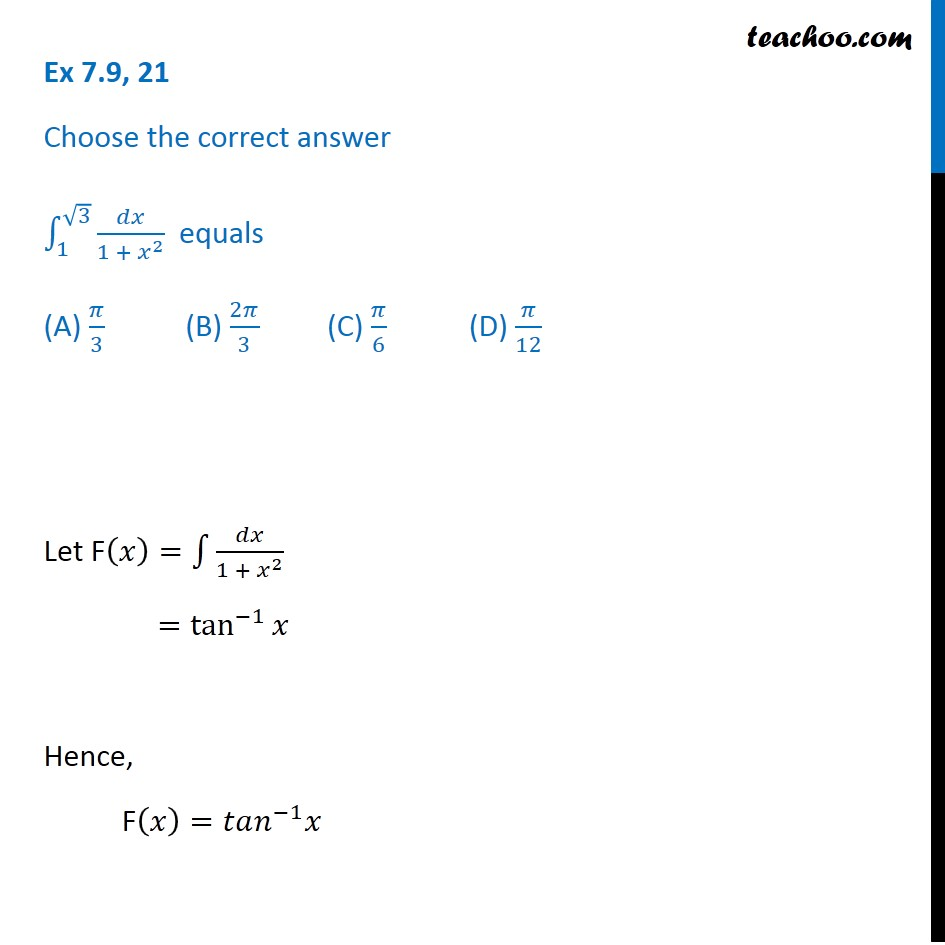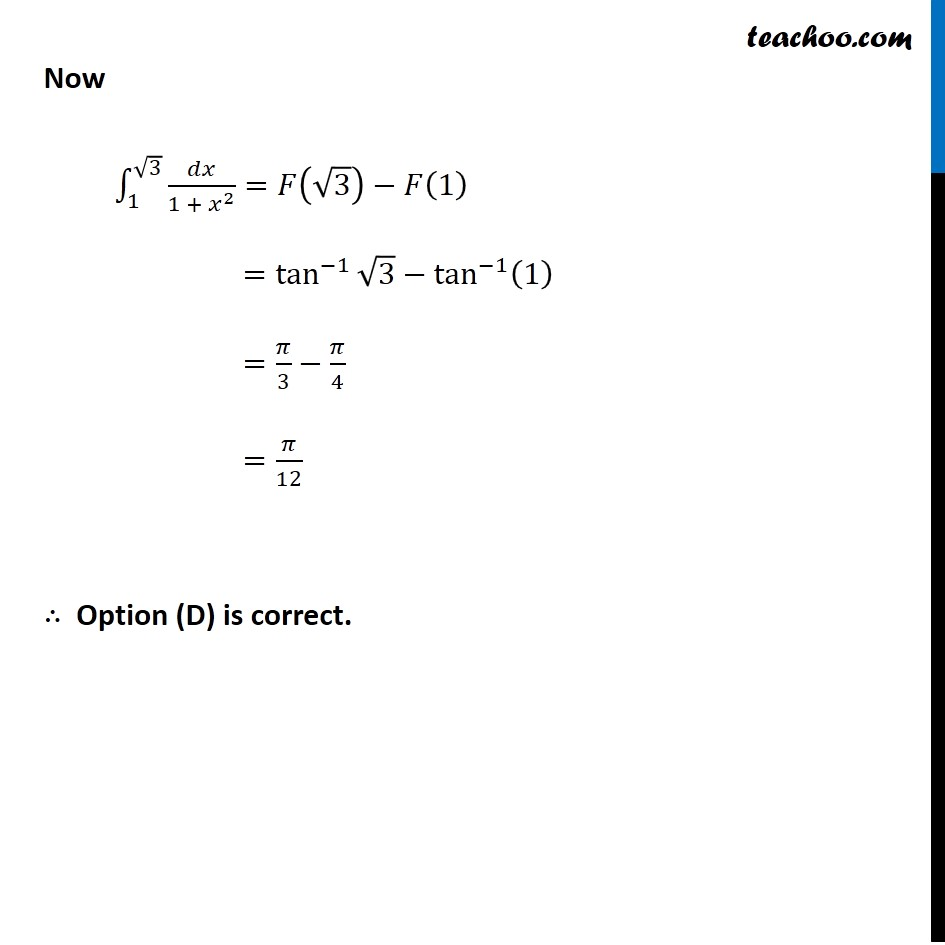Ex 7.8

Chapter 7 Class 12 Integrals
Serial order wiseLearn in your speed, with individual attention - Teachoo Maths 1-on-1 Class

### Transcript

Ex 7.8, 21 Choose the correct answer ∫_1^(√3)▒𝑑𝑥/(1 + 𝑥^2 ) equals (A) 𝜋/3 (B) 2𝜋/3 (C) 𝜋/6 (D) 𝜋/12 Let F(𝑥)=∫1▒𝑑𝑥/(1 + 𝑥^2 ) =tan^(−1)⁡𝑥 Hence, F(𝑥)=𝑡𝑎𝑛^(−1) 𝑥 Now ∫_1^(√3)▒〖𝑑𝑥/(1 + 𝑥^2 )=𝐹(√3)−𝐹(1) 〗 =tan^(−1)⁡〖√3−tan^(−1)⁡(1) 〗 =𝜋/3−𝜋/4 =𝜋/12 ∴ Option (D) is correct.